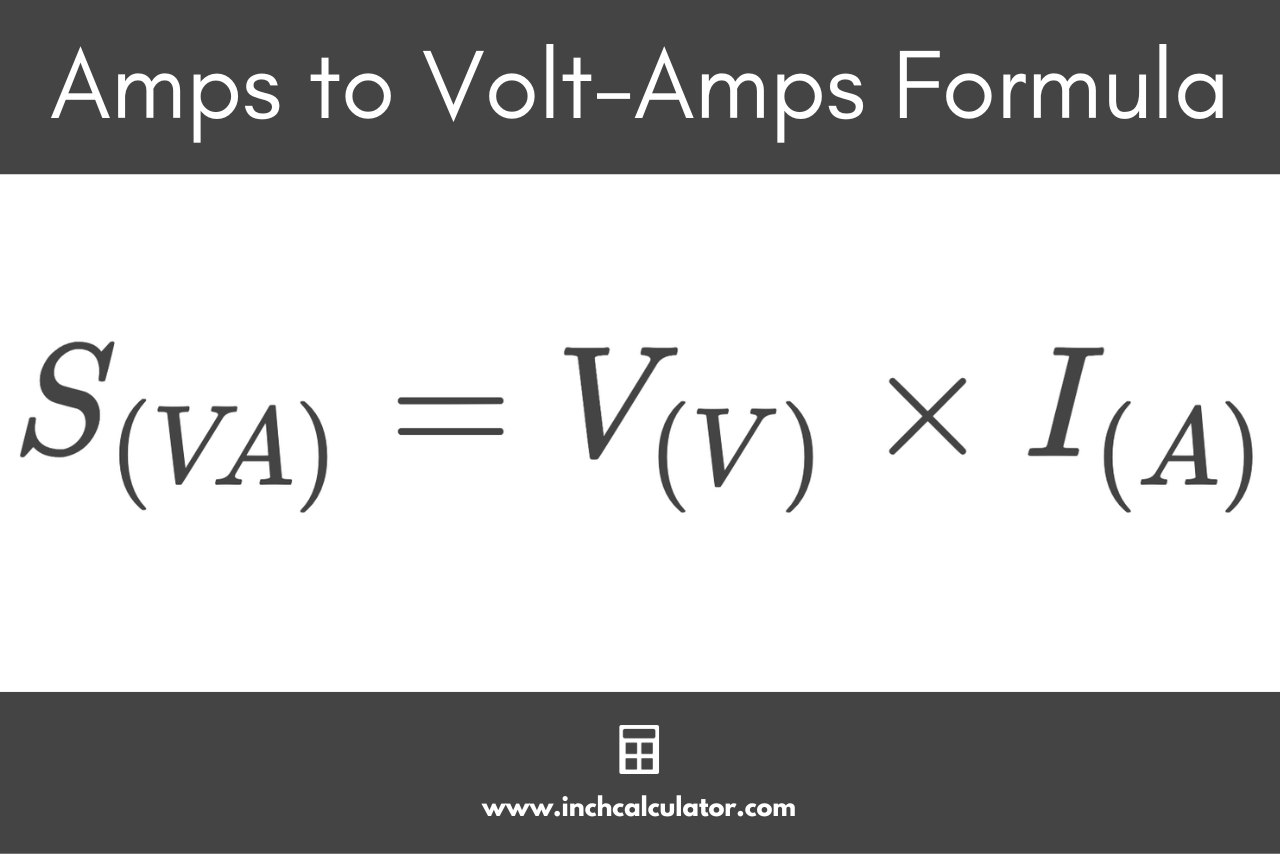# Amps to Volt-Amps (VA) Conversion Calculator

Convert amps to volt-amps by entering the current and voltage below. The calculator supports three-phase circuits, but standard single-phase circuits are set by default.

amps
volts

## Volt-Amps:

VA
Learn how we calculated this below

## How to Convert Amps to Volt-Amperes

Before converting amps to volt-amps it will be helpful to understand more about what these measurements are. An amp (A) is a measure of electric current, while a volt-ampere (VA) is a measure of apparent power in an electrical circuit.

Because this is a conversion from current and voltage to power, it’s possible to use the Watt’s Law power formula, which states that power is equal to the voltage times the current in an electrical circuit.
Power = Voltage × Current

By rearranging these variables with amps, volt-amps, and volts, the amps to volt-amps formula can be derived:

S(VA) = V(V) × I(A)

Thus, the apparent power S is equal to the voltage V times the current I in amps.Do you want to learn how to convert volt-amps to amps?

For example, let’s find the volt-amps for a single-phase circuit with 20 amps of current at 120 volts.

S(VA) = 120 V × 20 A
S(VA) = 2,400 VA

So, a 120 volt circuit with 20 amps of current should have 2,400 volt-amps of apparent power.

### How to Convert Amps to Volt-amps for a Three-Phase Circuit

Converting amps to volt-amps is slightly different for three-phase electrical circuits. A modified formula will be needed for the calculation.

S(VA) = √3 × VL-L(V) × I(A)

For three-phase circuits, the apparent power S in volt-amps is equal to the square root of 3 times the line to line voltage V times to current I in amps.

For example, let’s find the amps for a 480 volt three-phase electrical circuit with 60 amps of current.

S(VA) = √3 × 480 V × 60 A
S(VA) = 49,883 VA

Thus, a 480 volt three-phase electrical circuit with 60 amps of current has an apparent power of 49,883 volt-amperes.